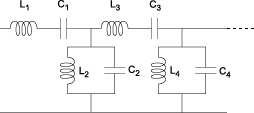# rfckt.lcbandpasstee

Bandpass tee filter

## Description

Use the `lcbandpasstee` class to represent a bandpass tee filter as a network of inductors and capacitors.

The LC bandpass tee network object is a 2-port network as shown in the following circuit diagram.In the diagram, [L1, L2, L3, L4, ...] is the value of the `'L'` object property, and [C1, C2, C3, C4, ...] is the value of the `'C'` object property.

## Creation

### Syntax

``h = rfckt.lcbandpasstee``
``h = rfckt.lcbandpasstee('Property1',value1,'Property2',value2,...)``

### Description

example

````h = rfckt.lcbandpasstee`returns an LC bandpass tee network object whose properties all have their default values.```
````h = rfckt.lcbandpasstee('Property1',value1,'Property2',value2,...)` sets properties using one or more name-value pairs. You can specify multiple name-value pairs. Enclose each property name in a quote```

## Properties

expand all

Computed S-parameters, noise figure, OIP3, and group delay values, specified as rfdata.data object. The values are computed over the specified frequency range. By default, this property is empty.

Data Types: `function_handle`

Capacitance value from source to load of all capacitors in the network, specified as a positive vector in farads. The length of the capacitance vector must be equal to the length of the vector you provide for `'L'`. The default value is ```[0.0186e-10, 0.1716e-10, 0.0186e-10]```.

Data Types: `double`

Inductance value from source to load of all inductors in the network, specified as a positive vector in henries. The length of the inductance vector must be equal to the length of the vector you provide for `'C'`. The default value is ```[0.2781e-7, 0.0301e-7, 0.2781e-7]```.

Data Types: `double`

Object name, specified as a `1-by-N` character array. This is a read-only property.

Data Types: `char`

Number of ports, specified as a positive integer. This is a read-only property. The default value is `2`.

Data Types: `double`

## Object Functions

 `analyze` Analyze RFCKT object in frequency domain `calculate` Calculate specified parameters for rfckt objects or rfdata objects `circle` Draw circles on Smith Chart `extract` Extract specified network parameters from rfckt object or data object `listformat` List valid formats for specified circuit object parameter `listparam` List valid parameters for specified circuit object `loglog` Plot specified circuit object parameters using log-log scale `plot` Plot circuit object parameters on X-Y plane `plotyy` Plot parameters of RF circuit or RF data on X-Y plane with two Y-axes `getop` Display operating conditions `polar` Plot specified object parameters on polar coordinates `semilogx` Plot RF circuit object parameters using log scale for x-axis `semilogy` Plot RF circuit object parameters using log scale for y-axis `smith` Plot circuit object parameters on Smith chart `write` Write RF data from circuit or data object to file `getz0` Calculate characteristic impedance of RFCKT transmission line object `read` Read RF data from file to new or existing circuit or data object `restore` Restore data to original frequencies `getop` Display operating conditions `groupdelay` Group delay of S-parameter object or RF filter object or RF Toolbox circuit object

## Examples

collapse all

Create a LC Bandpass Tee Filter using `rfckt.lcbandpasstee`.

`filter = rfckt.lcbandpasstee('C',[1e-12 4e-12],'L',[2e-9 2.5e-9])`
```filter = rfckt.lcbandpasstee with properties: L: [2x1 double] C: [2x1 double] nPort: 2 AnalyzedResult: [] Name: 'LC Bandpass Tee' ```

 Ludwig, R. and P. Bretchko, RF Circuit Design: Theory and Applications, Prentice-Hall, 2000.

 Zverev, A.I., Handbook of Filter Synthesis, John Wiley & Sons, 1967.